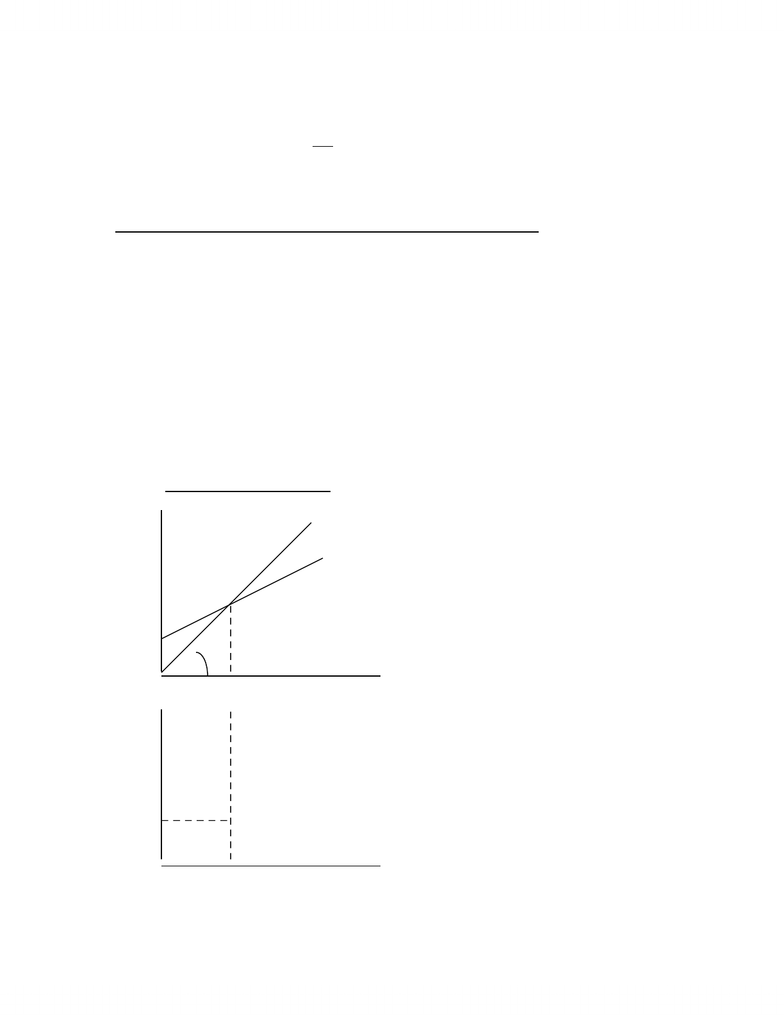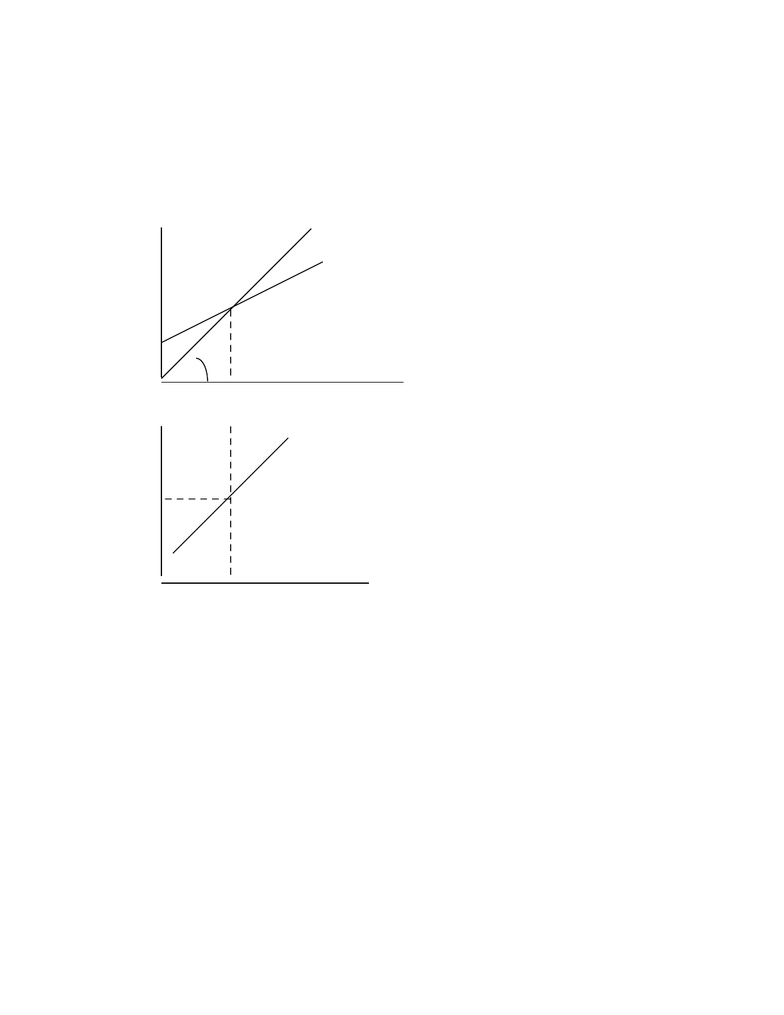# Chapter 16 Notes

40 views15 pagesChapter 16: Output and the Exchange Rate in the Short Run
Determinants of Aggregate Demand (AD) in an Open Economy
There are 4 sources of AD in an open economy:
1) Consumption (C) – C is determined by disposable income, Y – T.
C = C(Y – T) = Autonomous C + MPC×(Y –T)
2) Investment (I) – I is determined autonomously.
3) Government spending (G) – G is determined autonomously.
4) Net export/CA – CA is determined by (Y – T) and q.
CA = CA(Y – T, q) = Autonomous CA – MPIM × (Y –T) + CA1×q
( )
=+++=
TY ,
P
*
P
EqCAGITY CD
( )
( )
( ) ( ) ( )
)
P
*
P
E q,G,I,T,YD(D
+
=
+
++
=
How Output is Determine in the Short Run
ECMC61 – Chapter 16 1
www.notesolution.com
Unlock document

This preview shows pages 1-3 of the document.
Unlock all 15 pages and 3 million more documents.Output market equilibrium is given by AD = AS = Output (Y).
( ) ( ) ( ) ( ) ( )
)
P
*
P
E,G,I,T,YD(Y
+
+
++
=
In the short run, P are P* are held fixed.
Output Market Equilibrium in the Short Run: The DD Schedule
The DD schedule: it shows the relationship between output and the exchange rate for
which the output market is in short-run equilibrium.
Deriving the DD Schedule
Along the D = Y line, the output market is in equilibrium.
Along any aggregate demand curve (D), autonomous C, I, G, T, autonomous CA, E,
P and P* are held constant.
Keynesian Cross Diagram
D Y = D
D(E0)
A
45o
Y0 Y
E
E0 A
Y0 Y
ECMC61 – Chapter 16 2
www.notesolution.com
Unlock document

This preview shows pages 1-3 of the document.
Unlock all 15 pages and 3 million more documents.Factors that Shift the DD Schedule
Changes in Y, T, I, G, and q affect AD. Since the DD schedule is displayed in the (Y,
E) space, so factors that affect AD other than E and Y will shift the curve.
1) A change in G:
D Y = D
D(G0,E0)
A
45o
Y0 Y
E
DD(G0)
E0 A
Y
Y0
ECMC61 – Chapter 16 3
www.notesolution.com
Unlock document

This preview shows pages 1-3 of the document.
Unlock all 15 pages and 3 million more documents.

# Get access

\$10 USD/m
Billed \$120 USD annually
Homework Help
Class Notes
Textbook Notes